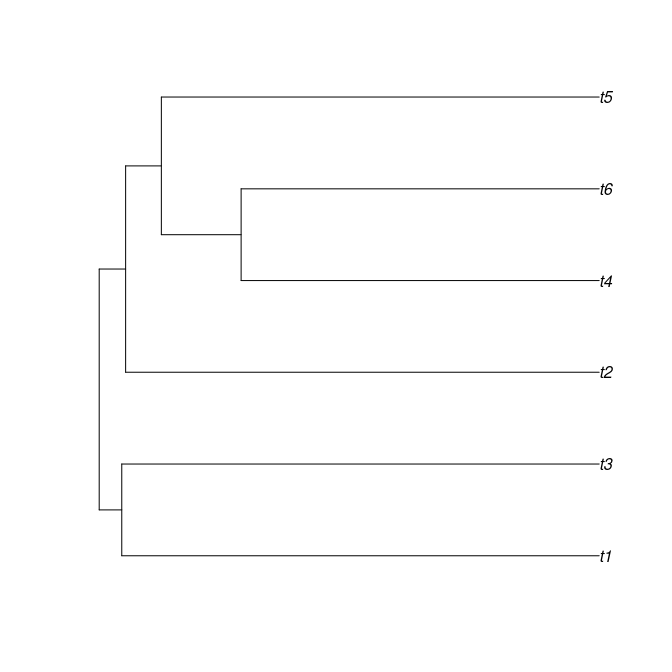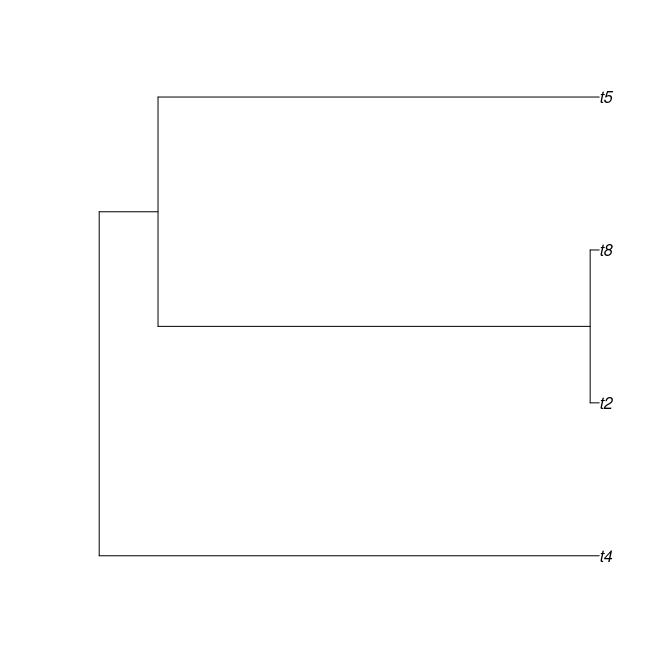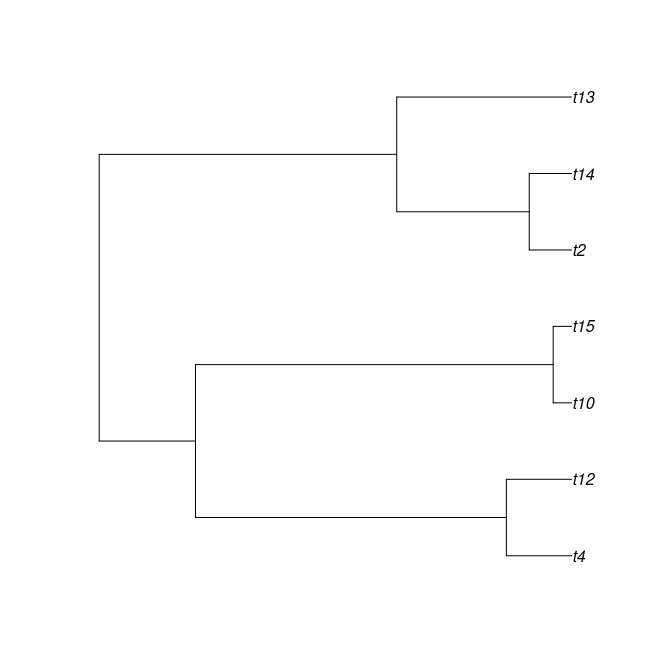# Experiment

## Introduction

This vignette shows how to use pirouette in a scientific experiment.

library(pirouette)

Remember the main question pirouette helps to answer:

Would we know the true phylogeny in nature, how well would we be able to infer it from a DNA alignment?

In this vignette, we investigate the effect of extintion rate in inferring a phylogeny from a (constant rate) birth-death process. We expect that inference is better if there is more information to work on. For example, when there are more taxa. But extinctions do not only decrease the number of taxa, also the information when these extinct taxa cam into exinstence is lost. As extinctions lower the amount of information, we predict a higher inference error for higher extinction rates.

Our experimental setup will not be the best setup. A good experimental setup will have, among others, more replicates, longer MCMC runs and a more equal comparison.

## Setup

We specify the extinction rates we will investigate:

ext_rates <- seq(0.0, 0.4, length.out = 3)

For a speciation rate, we use one value:

spec_rate <- 1.0

Now, per extinction rate, we simulate one phylogeny:

set.seed(42)
crown_age <- 4.0
n_taxa <- 6
phylogenies <- list()
for (i in seq_along(ext_rates)) {
ext_rate <- ext_rates[i]
phylogeny <- create_exemplary_dd_tree(
n_taxa = n_taxa,
crown_age = crown_age,
extinction_rate = ext_rate
)
phylogenies[[i]] <- phylogeny
}
ape::plot.phylo(phylogenies[])ape::plot.phylo(phylogenies[])ape::plot.phylo(phylogenies[])Now, per phylogeny, we estimate the error BEAST2 makes:

alignment_params <- create_alignment_params(
root_sequence = create_blocked_dna(length = 20)
)
experiment <- create_experiment(
inference_conditions = create_inference_conditions(
model_type = "generative",
run_if = "always",
do_measure_evidence = FALSE
),
inference_model = create_inference_model(
tree_prior = create_bd_tree_prior(),
mcmc = create_test_mcmc()
)
)
experiments <- list(experiment)
pir_params <- create_pir_params(
alignment_params = alignment_params,
experiments = experiments
)
errors <- list()
if (rappdirs::app_dir()$os != "win" && beastier::is_beast2_installed()) { for (i in seq_along(ext_rates)) { phylogeny <- phylogenies[[i]] df <- pirouette::pir_run( phylogeny = phylogeny, pir_params = pir_params ) errors[[i]] <- df } } Now, we put all data in one data frame: if (rappdirs::app_dir()$os != "win" && beastier::is_beast2_installed()) {
first_error_col <- which(colnames(errors[]) == "error_1")
last_error_col <- ncol(errors[])
n_errors <- 1 + last_error_col - first_error_col
df <- data.frame(
ext_rate = as.factor(rep(ext_rates, each = n_errors)),
idx = seq(1, n_errors),
error = NA
)

for (i in seq_along(ext_rates)) {
this_df <- errors[[i]]
nltts <- this_df[1, first_error_col:last_error_col]
to_row_index <- 1 + (i * n_errors) - n_errors
df$error[to_row_index:(to_row_index + n_errors - 1)] <- t(as.numeric(nltts)) } } Plotting it: if (rappdirs::app_dir()$os != "win" && beastier::is_beast2_installed()) {
ggplot2::ggplot(
df, ggplot2::aes(x = ext_rate, y = error)
) + ggplot2::geom_boxplot()
ggplot2::ggplot(
df, ggplot2::aes(x = ext_rate, y = error)
) + ggplot2::geom_violin()
}